Courses

# RD Sharma Solutions -Ex-7.2, Decimals, Class 6, Maths Class 6 Notes | EduRev

## RD Sharma Solutions for Class 6 Mathematics

Created by: Abhishek Kapoor

## Class 6 : RD Sharma Solutions -Ex-7.2, Decimals, Class 6, Maths Class 6 Notes | EduRev

The document RD Sharma Solutions -Ex-7.2, Decimals, Class 6, Maths Class 6 Notes | EduRev is a part of the Class 6 Course RD Sharma Solutions for Class 6 Mathematics.
All you need of Class 6 at this link: Class 6

Question 1

i) Three tenths

Solution: 3/10= 0.3

ii) Two ones and five tenths

Solution: 2+5/10=2.5

iii) Thirty and one tenths

Solution: 30+1/10=30.1

iv) Twenty two and six tenths

Solution:22+6/10=22.6

v) One hundred , two ones and three tenths

Solution: 100+2+3/10=102.3

Question 2

i) 30+6+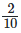Solution: We have 3 tens , 6 ones and 2 tenths. Therefore , the decimal is 36.2

ii) 700+5+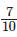Solution: We have 7 hundreds, 5 ones and 7 tenths. Therefore the decimal is 705.7

iii) 100+60+5+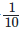Solution: We have 2 hundreds, 6 tens, 5 ones and 1 tenths. Therefore the decimal is 265.1

iv) 200+70+9+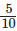Solution: We have 2 hundreds ,7 tens, 9 ones and 5 tenths. Therefore , the decimal is 279.5

Question 3

i)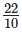Solution: Since the denominator is ten , the decimal is 2.2

ii)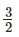Solution: Making the denominator 10, we have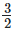3(52)(5) =1510 = 1.5

iii)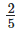Solution: Making the denominator 10 , we have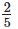2(25)(2) =410 =0.4

Question 4

i)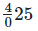Solution: To write in decimal, we need to make the denominator 10 by multiplying it by a number. But, to maintain the value of the fraction, we should also multiply the numerator by the same number. Thus, we get

= 40+2(25)(2) = 40+410 = 40.4

ii)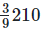Solution: 39210 = 39+210

Here, the denominator is 10 .

Therefore, the decimal is 39.2

iii)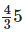Solution: 435=4+35

To write in decimal, we need the denominator by 10 by multiplying it by a number . but, to maintain the value of the fraction , we should also multiply the numerator by the same number. Thus we get ,

=4+3(3)(25)(2)

=4+610=4.6

iv)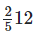Solution: 2512=25+12

To write in decimal, we need to make the denominator 10 by multiplying it by a number. But, to maintain the value of the fraction, we should also multiply the numerator by the same number. Thus, we get

=25+1(52)(5)

=25+510=25.5

Question 5

i) 3.8

Solution:
=3+8 tenths

= 3+810

= 3(1010)+810 = 3010+810 = 3810 = 195

ii) 21.2
Solution:

=21+2 tenths

=21+210= 21(1010)+210 = 21010+210 = 21210 = 1065

iii) 6.4
Solution:

= 6+4 tenths

= 6+410

= 6(1010) +410 = 6010+410 = 325

iv) 1
Solution:

Since the only number after the decimal is 0, the fraction is 1

Question 6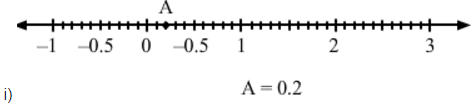Solution: 0.2

ii)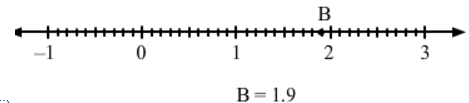Solution: 1.9

iii)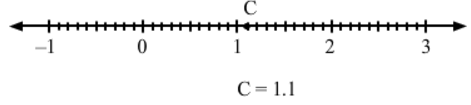Solution: 1.1

iv)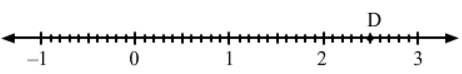Solution: 2.5

Question 7

i) 0.8 is between the two whole numbers 0 and 1

Solution: 0.8 is 8 units from 0 and 2 units from 1, it is nearer to 1

ii) 5.1 is between the two whole number 5 and 6.

Solution: 5.1 is 1 unit from 5 and 9 units from 6, it is nearer to 5

iii) 2.6 is between 2 and 3

Solution: 2.6 is 6 units from 2 and 4 units from 3 , it is nearer to 3

iv) 6.4 is between 6 and 7

Solution: 6.4 is 4 units from 6 and 6 units from 7, it is nearer to 6
v) 4.9 is between 4 and 5

Solution4.9 is 9 units from 4 and 1 unit from 5, it is nearer to 5.

Question 8

Write the decimal number represented by the points on thye given number line A,B,C,D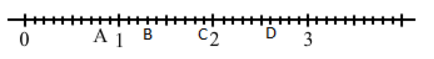Solution:
A) 0.8 , since A is at the eighth place between 0 and 1

B) 1.3 ,since B is at the third place between 1 and 2

C) 1.9 , since C is at the ninth place between 1 and 2

D) 2.6 , since D is at the sixth place between 2 and 3

Disclaimer: the image given in the book is not consistent; as the number of periods between 0 and 1 is ten but the number of periods between 1 and 2 are seven. So, ignoring the position of the given numbers 1, 2 and 3 . it has been assumed that there are ten periods between every two consecutive numbers starting from the first point taken as zero.

103 docs

,

,

,

,

,

,

,

,

,

,

,

,

,

,

,

,

,

,

,

,

,

,

,

,

,

,

,

,

,

,

;